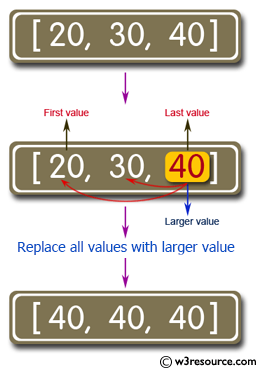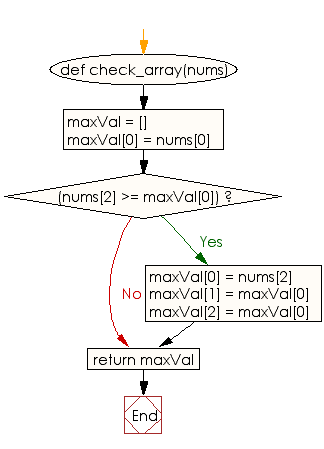﻿ Ruby Array exercises: Find the larger between the first and last elements of an given array of integers - w3resource# Ruby Array Exercises: Find the larger between the first and last elements of a given array of integers

## Ruby Array: Exercise-13 with Solution

Write a Ruby program to find the larger between the first and last elements of a given array of integers of length 3. Replace all the other values to be that value. Return the changed array.Ruby Code:

``````def check_array(nums)
maxVal = []
maxVal = nums
if(nums >= maxVal)
maxVal = nums
maxVal = maxVal
maxVal = maxVal
end
return maxVal
end
print check_array([1, 2, 5]),"\n"
print check_array([1, 2, 3]),"\n"
print check_array([1, 2, 4])
``````

Output:

```[5, 5, 5]
[3, 3, 3]
[4, 4, 4]
```

Flowchart:Ruby Code Editor: